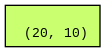# Gradient not computed for nn.Parameter

I have a model with a linear layer and I wish to multiply the linear layer weights element-wise with a torch.nn.Parameter at every forward pass. I tried it the the following way but this not compute a gradient for the parameter.

class LinearModel(nn.Module):

``````def __init__(self, args):
super(LinearModel, self).__init__()
self.linear1 = nn.Linear(10, 20)

def forward(self, x)
return self.linear1(x)
``````

The loss is squared loss. When I print self.mask.grad, it shows None. Where am I going wrong?

Your code should not even be executable and fails with:

``````lin = LinearModel(0)
x = torch.randn(1, 10)
out = lin(x)
# TypeError: cannot assign 'torch.FloatTensor' as parameter 'weight' (torch.nn.Parameter or None expected)
``````

since you are trying to assign a tensor to a parameter.
In case you want to manipulate the `linear1.weight` parameters inplace before executing the forward pass, wrap it into a `torch.no_grad()` guard or use the functional API via:

``````class LinearModel(nn.Module):
def __init__(self):
super(LinearModel, self).__init__()
self.linear1 = nn.Linear(10, 20)

def forward(self, x):
return F.linear(x, weight, self.linear1.bias)

lin = LinearModel()
x = torch.randn(1, 10)
out = lin(x)
out.mean().backward()
``````

Thank you! Sorry, I pasted an outdated code (I tried `self.linear1.weight = nn.Parameter(self.linear1.weight*self.mask`) but that gave None as the gradient for `mask`.

Can you please show how I can do the same using `torch.no_grad()` as you earlier suggested?

Hi,
That is expected.
As you are essentially creating a new parameter with this line of code and that would be a leaf tensor (with its `grad_fn=None`).
This means `mask` is no more present in the computation graph of `linear1.weight` or `out` and hence does not get its `grad` populated.

See the following code - I have used torchviz to visualize the comp graphs :

``````import torch
import torch.nn as nn

class LinearModel(nn.Module):
def __init__(self):
super(LinearModel, self).__init__()
self.linear1 = nn.Linear(10, 20)

def forward(self, x):
print(self.linear1.weight.is_leaf) # True
make_dot(self.linear1.weight).render("weight", format="png") # image 1
return self.linear1(x)

lin = LinearModel()
x = torch.randn(1, 10)
out = lin(x)
make_dot(out).render("output", format="png") # image 2
out.mean().backward()

``````

Now, if you see image 1 below it is a leaf with no graph associated with it. Hence, mask isn’t present in both graphs anymore.

image 1 (linear1.weight)image 2 (out)
The top two blue nodes correspond to the bias and the weight term of the linear1 layer.Thank you! Is there a way I can do this without creating a new module? I don’t know how to do this with `torch.no_grad()` as suggested earlier. I do want the gradient to be computed for `mask`.

Is there any specific reason not to use the code posted by @ptrblck above?

With torch.no_grad, you could definitely modify the weight of the linear1 layer by replacing `self.linear1.weight = nn.Parameter(self.linear1.weight*self.mask)` with -

``````with torch.no_grad():